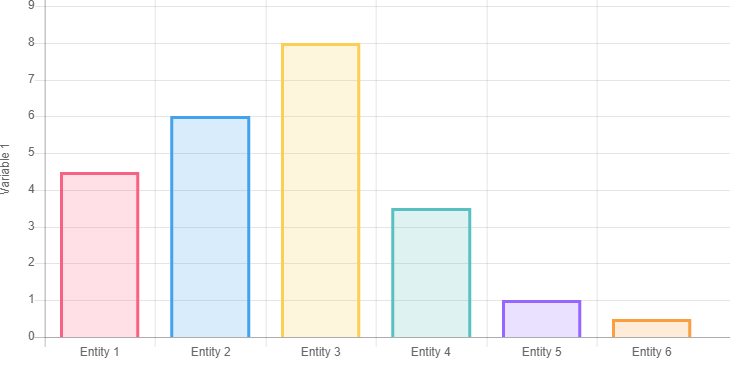Bar charts are a very common type of chart used primarily to visually compare variables.

A WordPress administrator that needs bar charts in its post has both the option to install a chart plugin or upload images generated with external programs.

## Create bar charts with a WordPress plugin

Using a chart plugin gives you many advantages:

• The charts created with a plugin can be interactive and thus have animations, active tooltips, and more
• You can at any time modify the chart if changes are required
• Charts generated with the HTML Canvas or with dynamic SVG are lighter than charts uploaded as raster images
• You have a unique and centralized menu to handle all the charts of the website

This tutorial will use UberChart, a premium WordPress plugin based on the Chart.js JavaScript library, to create all the bar charts.

The UberChart plugin can easily generate all the variations of a bar chart:

### Bar chart

This section covers the simplest type of bar chart.

#### Vertical bar chart

To create a vertical bar chart, proceed as follows:

• Load the “Bar chart with a single dataset” model
• Save the chartA generic vertical bar chart with a category scale in the x axis, a linear scale in the y axis and dummy data.

#### Horizontal Bar Chart

To create a horizontal bar chart, proceed as follows:

• Load the “Horizontal bar chart with a single dataset” model
• Save the chart

### Grouped Bar Chart

When each category of the bar chart can be split into multiple subcategories, or when the primary category represents a time interval, grouped bar charts can be used.

#### Vertical Grouped Bar Chart

To create a vertical grouped bar chart, proceed as follows:

• Load the “Bar chart with a single dataset” model
• Increase the number of datasets to more than one with the Rows field
• Save the chart

#### Horizontal Grouped Bar Chart

To create a horizontal grouped bar chart, proceed as follows:

• Load the “Horizontal bar chart with multiple datasets” model
• Save the chart

### Stacked Bar Chart

A stacked bar chart is recommended when each category of a bar chart can be divided into multiple parts that form a total.

#### Vertical Stacked Bar Chart

Use this procedure to create a vertical stacked bar chart:

• Load the “Bar chart with multiple stacked datasets” model
• Save the chart

#### Horizontal Stacked Bar Chart

Use this procedure to create a horizontal stacked bar chart:

• Load the “Horizontal bar chart with multiple stacked datasets” model
• Save the chart

### Histogram

Histograms are used to represent the distribution of the same variable visually. In a histogram, the categories are numerical ranges, and the space used to separate the bars of a bar chart is not present.

#### Vertical Histogram

Create a vertical histogram with this procedure:

• Load the “Bar chart with a single dataset” model
• Set the categories with numerical ranges in the spreadsheet editor
• Set the X Scale Options -> Category Percentage and the X Scale Options -> Bar Percentage option to “1”
• Set the Border Width and the Hover Border Width of the dataset to “0”
• Save the chart

#### Horizontal histogram

Create a horizontal histogram with this procedure:

• Load the “Horizontal bar chart with a single dataset” model
• Set the categories with numerical ranges in the spreadsheet editor
• Set the Y Scale Options -> Category Percentage and the Y Scale Options -> Bar Percentage option to “1”
• Set the Border Width and the Hover Border Width of the dataset to “0”
• Save the chart